• Backprop sometimes has bugs. Gradient checking will check wheter backprop will eventually decreasing.....
• this will teach how 100% accurate backprop without bug....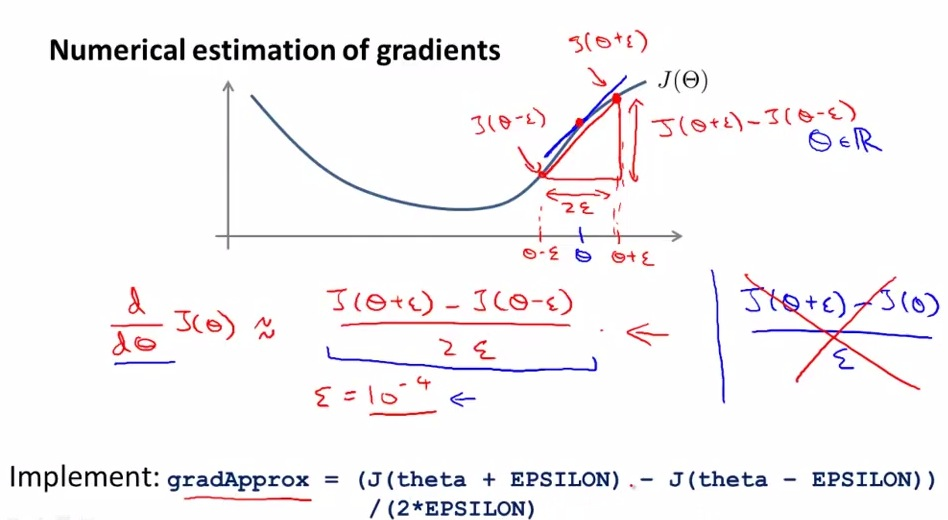• Takes an error based on epsilon
• Two sided difference will make better correction than one sided difference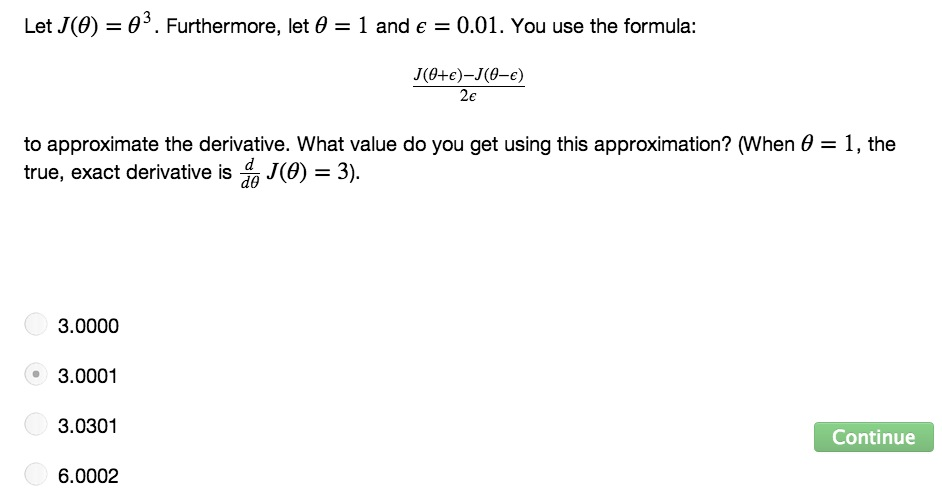• 3+10^-4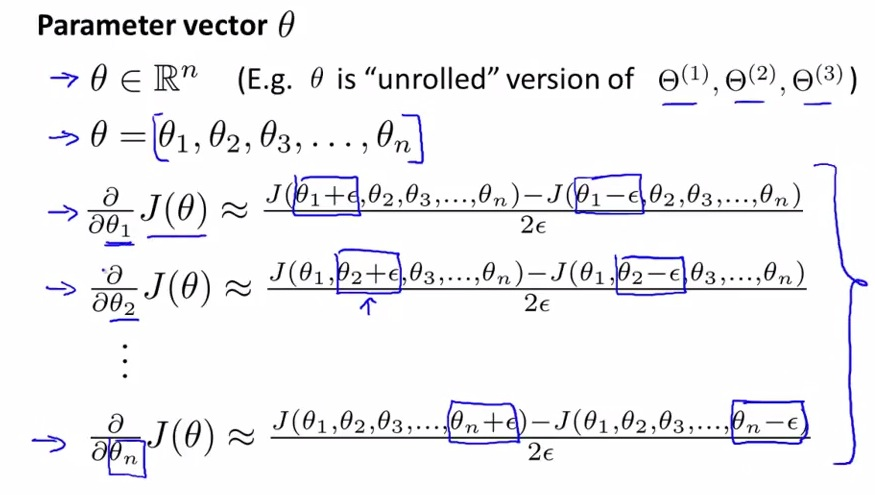• let theta be a matrix
• the epsilon will only be used to particular theta
• this way, the particular theta that being calculated will always be corrected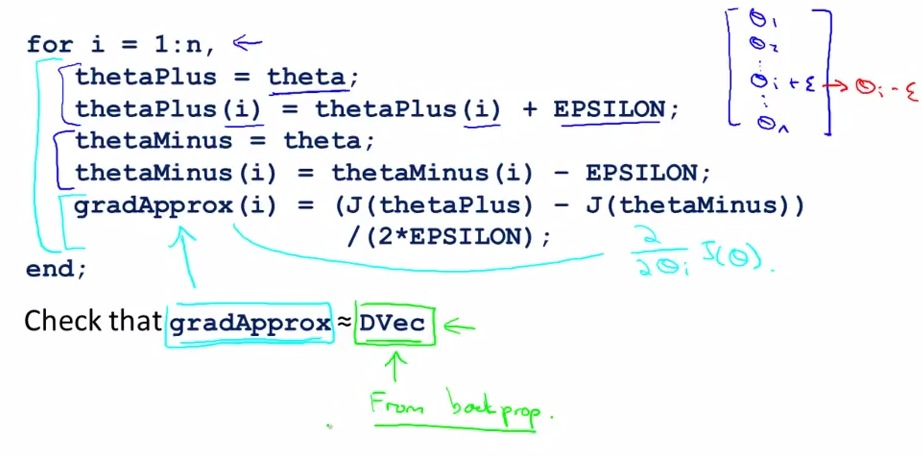• dvec is a partial derrivative from backpop that used as a cost function
• if the gradApprox is more or less same as Dvec, then the derrivative will surely output the right result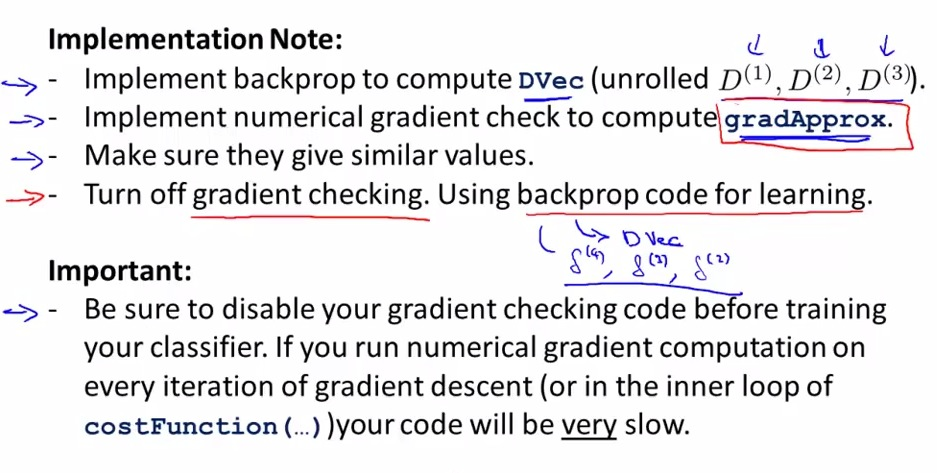• Here's the step on how to implement numerical gradient checking
• The important things is to be sure to shut gradient checking after checking the backprop. This is intended because gradient checking is computationally expensive
• Backprop code is much more efficient and faster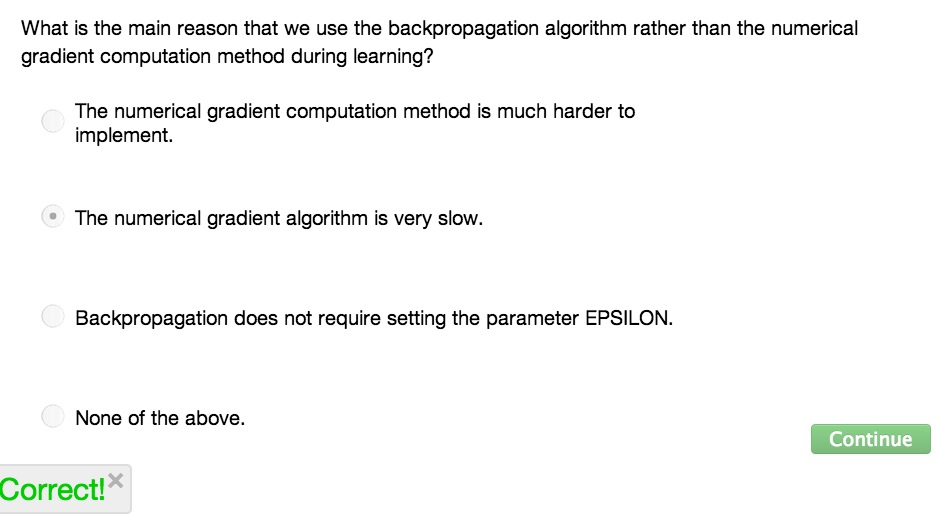• These method (gradient checking) is to makes sure the code that we made, is correctly decreasing from backprop value